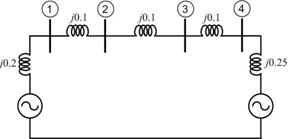## Construction of Sequence Network in Power System:

Before sections the Sequence Network of various Power System elements synchronous machines, transformers and lines have been given. Using these, the Construction of Sequence Network in Power System can be explained. To start with, the positive Sequence Network in Power System is constructed by examination of the one-line diagram of the system. It is to be noted that positive sequence voltages are present in synchronous machines (generators and motors) only.

The transition from positive sequence network to negative sequence network is straightforward. Since the positive and negative sequence imped­ances are identical for static elements (lines and transformers), the only change necessary in positive sequence network to obtain negative sequence network is in respect of synchronous machines.

Each machine is represented by its negative sequence impedance, the negative sequence voltage being zero.The reference bus for positive and negative sequence networks is the system neutral. Any impedance connected between a neutral and ground is not included in these sequence networks as neither of these sequence currents can flow in such an impedance.

Zero sequence subnetworks for various parts of a system can be easily combined to form complete zero sequence network. No voltage sources are present in the zero Sequence Network. Any impedance included in generator or transformer neutral becomes three times its value in a zero sequence network. Special care needs to be taken of transformers in respect of zero sequence network.

Scroll to Top